# CITIES_demo

#### 2023-07-27

library(cities)
library(dplyr)
library(tidyr)
library(plotly)
library(ggplot2)
library(ggthemes)

### Simulation Settings in CITIES (Figure 1 in publication)

The settings below simulates 50 datasets for a clinical trial with two arms; control and treatment over (0,24,48,72,96,120,144) weeks. The control arm has 120 subjects with a mean trajectory of (0,0,0,0,0,0,0) while the treatment arm has a mean trajectory of (0,0.1,0.2,0.4,0.6,0.8,1). The covariance structure for both arms are described using a partial autocorrelation of (-0.2, 0.4), each with a variance of 1. We supply no custom covariance and supply betas using the default covariance matrix provided, i.e. one continuous and one binary covariates. The lack of efficacy and excess efficacy functions are shown below, alongside the behaviors of discontinuity due to adminstrative reasons and adverse events.

Note the following

• First entry for all means must be the same, i.e. the baseline mean. In this case, this is 0
• Length of all means and timepoints must be the same
• The length of each beta vector must the same as the number of covariates
• prob_ae >= rate_dc_ae per arm
• z_l_loe <= z_u_loe
• z_l_ee <= z_u_ee
• pacf_vec values must be between -1 and 1, not inclusive
• reference_id must be either one of the arms. For example, if you have 4 arms, then reference_id should be either 1,2,3 or 4.
total_data = 50
reference_id = 1
threshold = NA
timepoints = c(0,24,48,72,96,120,144)
IR_display = TRUE

n_patient_ctrl = 120
n_patient_expt = 150
n_patient_vector = c(n_patient_ctrl, n_patient_expt)
n_total = sum(n_patient_vector)

mean_control = c(0,0,0,0,0,0,0)
mean_treatment = c(0,0.1,0.2,0.4,0.6,0.8,1)
mean_list = list(mean_control, mean_treatment)

sigma_ar_vec = c(1, 1)
pacf_list = list(c(-0.2, 0.4),
c(-0.2, 0.4))

beta_list = list(c(1.25, 1.25),
c(1.25, 1.25))
covariate_df = NA

# LoE & EE
up_good = "Up"
p_loe_max = 0.75
z_l_loe = -7
z_u_loe = -1
p_ee_max = 0.1
z_l_ee = 4
z_u_ee = 10

prob_ae_ctrl = 0.7
prob_ae_expt = 0.9
prob_ae = c(prob_ae_ctrl, prob_ae_expt)

rate_dc_ae_ctrl = 0.1
rate_dc_ae_expt = 0.1
rate_dc_ae = c(rate_dc_ae_ctrl, rate_dc_ae_expt)

starting_seed_val = 1
static_output = TRUE

### The Mean Treatment Response Settings tab in CITIES (Figure 2 in publication)

mean_out = plot_means(n_patient_vector = n_patient_vector,
timepoints = timepoints,
pacf_list = pacf_list,
sigma_ar_vec = sigma_ar_vec,
mean_list = mean_list,
beta_list = beta_list,
reference_id = reference_id,
seed_val = starting_seed_val,
total_data = total_data,
threshold = threshold,
covariate_df = covariate_df,
static_output = static_output)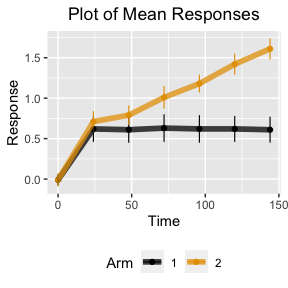### The LoE & EE tab in CITIES (Figure 3 in publication)

plot_loe_ee (mean_list = mean_list,
ref_grp = reference_id,
stdev_vec = sigma_ar_vec,
p_loe_max = p_loe_max,
z_l_loe = z_l_loe,
z_u_loe = z_u_loe,
p_ee_max = p_ee_max,
z_l_ee = z_l_ee,
z_u_ee = z_u_ee,
up_good = up_good,
greyscale = FALSE,
static_output = TRUE)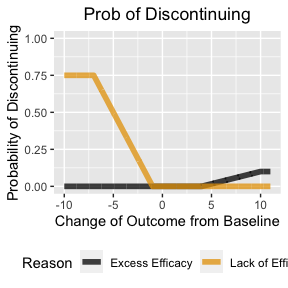### Treatment effect profiles for the all six estimands in CITIES (Figure 6 in publication)

data_out = data_generator_loop(n_patient_vector,
p_loe_max,
z_l_loe,
z_u_loe,
p_ee_max,
z_l_ee,
z_u_ee,
timepoints,
pacf_list,
sigma_ar_vec,
mean_list,
beta_list,
rate_dc_ae,
prob_ae,
starting_seed_val,
reference_id,
plot_po = FALSE,
up_good,
threshold,
total_data,
covariate_df)

estimates_out = plot_estimates(data_out = data_out,
total_data = total_data,
timepoints = timepoints,
reference_id = reference_id,
IR_display = IR_display,
normal_output = TRUE,
static_output = static_output)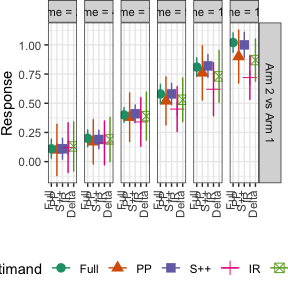### Treatment effect profiles for the all six estimands in CITIES (Table 1 in publication)

display_table = estimates_out %>%
mutate(mean_se = paste0(mean, " (", round(se, 2) , ")")) %>%
dplyr::select(-se, -Arm, -mean) %>%
pivot_wider(names_from = Estimand, values_from = mean_se)
display_table[,c(1,2,5,3,4,6,7)]
## # A tibble: 6 × 7
##   name  Timepoints Full        PP          S++       IR          Delta
##   <chr>      <dbl> <chr>       <chr>       <chr>       <chr>       <chr>
## 1 1             24 0.11 (0.08) 0.1 (0.22)  0.11 (0.09) 0.12 (0.21) 0.13 (0.21)
## 2 2             48 0.2 (0.07)  0.17 (0.19) 0.19 (0.08) 0.16 (0.19) 0.19 (0.19)
## 3 3             72 0.4 (0.06)  0.38 (0.21) 0.41 (0.08) 0.34 (0.21) 0.39 (0.21)
## 4 4             96 0.58 (0.08) 0.52 (0.21) 0.58 (0.09) 0.45 (0.19) 0.53 (0.19)
## 5 5            120 0.81 (0.08) 0.76 (0.23) 0.82 (0.1)  0.62 (0.23) 0.73 (0.22)
## 6 6            144 1.02 (0.09) 0.9 (0.23)  1 (0.11)    0.72 (0.19) 0.87 (0.18)

### Percentages of treatment discontinuations, and the corresponding ICEs in CITIES (Figure 7 in publication)

dc_out = plot_dc(data_out = data_out,
total_data = total_data,
timepoints = timepoints,
static_output = static_output)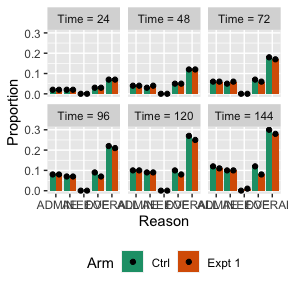dc_out1 = dc_out %>%
ungroup() %>%
filter(Timepoints == max(timepoints),
Reason != "OVERALL") %>%
select(Arm, Reason, Value) %>%
pivot_wider(names_from = Arm,
values_from = Value) %>%
arrange(factor(Reason, levels = c("AE", "LOE", "EE", "ADMIN")))
rbind(dc_out1,c("OVERALL",colSums(dc_out1[,-1])))
## # A tibble: 5 × 3
##   Reason  Ctrl  Expt 1
##   <chr>   <chr> <chr>
## 1 AE      0.1   0.1
## 2 LOE     0.12  0.08
## 3 EE      0     0.01
## 5 OVERALL 0.34  0.3

### Simulation Settings in CITIES for the Diabetes case study (Figure S1 in publication)

total_data = 100
reference_id = 1
threshold = NA
timepoints = c(0,6,12,18,26)
IR_display = FALSE

n_patient_ctrl = 195
n_patient_expt = 192
n_patient_vector = c(n_patient_ctrl, n_patient_expt)
n_total = sum(n_patient_vector)

mean_control = c(8, 8, 7.98, 7.97, 7.94)
mean_treatment = c(8, 7.45, 7.26, 7.21, 7.16)
mean_list = list(mean_control, mean_treatment)

sigma_ar_vec = c(0.8, 0.8)
pacf_list = c(0.5, 0.5)

beta_list = NA
covariate_df = NA

# LoE & EE
up_good = "Down"
p_loe_max = 0.25
z_l_loe = 1
z_u_loe = 4
p_ee_max = 0
z_l_ee = 0
z_u_ee = 0

p_admin_ctrl = 0.03 #(2+3+1+15+1+4)/192 = 0.1354167
p_admin_expt = 0.02 #(5+2+9+3)/197 = 0.0964467

prob_ae_ctrl = 0.53 #101/192
prob_ae_expt = 0.6 #118/197
prob_ae = c(prob_ae_ctrl, prob_ae_expt)

rate_dc_ae_ctrl = 0.01 # 2/192
rate_dc_ae_expt = 0.02 # 4/197
rate_dc_ae = c(rate_dc_ae_ctrl, rate_dc_ae_expt)

starting_seed_val = 1
static_output = TRUE

### The Mean Treatment Response Settings tab in CITIES for the Diabetes case study (Figure S2 in publication)

mean_out = plot_means(n_patient_vector = n_patient_vector,
timepoints = timepoints,
pacf_list = pacf_list,
sigma_ar_vec = sigma_ar_vec,
mean_list = mean_list,
beta_list = beta_list,
reference_id = reference_id,
seed_val = starting_seed_val,
total_data = total_data,
threshold = threshold,
covariate_df = covariate_df,
static_output = static_output)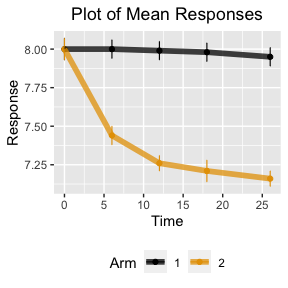### The LoE & EE tab in CITIES for the Diabetes case study (Figure S3 in publication)

plot_loe_ee (mean_list = mean_list,
ref_grp = reference_id,
stdev_vec = sigma_ar_vec,
p_loe_max = p_loe_max,
z_l_loe = z_l_loe,
z_u_loe = z_u_loe,
p_ee_max = p_ee_max,
z_l_ee = z_l_ee,
z_u_ee = z_u_ee,
up_good = up_good,
greyscale = FALSE,
static_output = TRUE)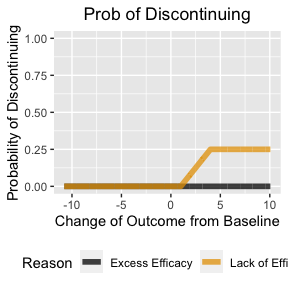### Treatment effect profiles for the three estimands in CITIES for the Diabetes case study (Figure S5 in publication)

data_out = data_generator_loop(n_patient_vector,
p_loe_max,
z_l_loe,
z_u_loe,
p_ee_max,
z_l_ee,
z_u_ee,
timepoints,
pacf_list,
sigma_ar_vec,
mean_list,
beta_list,
rate_dc_ae,
prob_ae,
starting_seed_val,
reference_id,
plot_po = FALSE,
up_good,
threshold,
total_data,
covariate_df)

estimates_out = plot_estimates(data_out = data_out,
total_data = total_data,
timepoints = timepoints,
reference_id = reference_id,
IR_display = IR_display,
normal_output = TRUE,
static_output = static_output)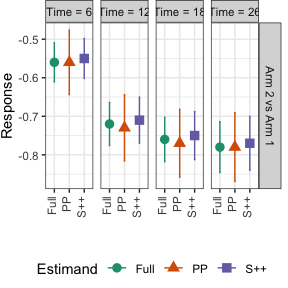### Treatment effect profiles for the three estimands in CITIES for the Diabetes case study (Table 3 in publication)

display_table = estimates_out %>%
mutate(mean_se = paste0(mean, " (", round(se, 2) , ")")) %>%
dplyr::select(-se, -Arm, -mean) %>%
pivot_wider(names_from = Estimand, values_from = mean_se)
display_table[,c(1,2,5,3,4)]
## # A tibble: 4 × 5
##   name  Timepoints Full         PP           S++
##   <chr>      <dbl> <chr>        <chr>        <chr>
## 1 1              6 -0.56 (0.05) -0.56 (0.08) -0.55 (0.05)
## 2 2             12 -0.72 (0.06) -0.73 (0.09) -0.71 (0.06)
## 3 3             18 -0.76 (0.06) -0.77 (0.09) -0.75 (0.06)
## 4 4             26 -0.78 (0.07) -0.78 (0.09) -0.77 (0.07)

### Percentages of treatment discontinuations, and the corresponding ICEs in CITIES for the Diabetes case study (Figure S6 in publication)

dc_out = plot_dc(data_out = data_out,
total_data = total_data,
timepoints = timepoints,
static_output = static_output)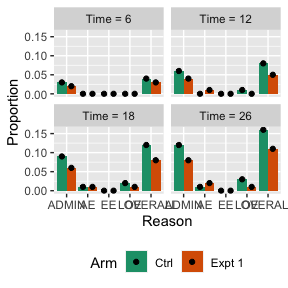### Percentages of treatment discontinuations, and the corresponding ICEs in CITIES for the Diabetes case study (Table 2 in publication)

dc_out1 = dc_out %>%
ungroup() %>%
filter(Timepoints == max(timepoints),
Reason != "OVERALL") %>%
select(Arm, Reason, Value) %>%
pivot_wider(names_from = Arm,
values_from = Value) %>%
arrange(factor(Reason, levels = c("AE", "LOE", "EE", "ADMIN")))
rbind(dc_out1,c("OVERALL",colSums(dc_out1[,-1])))
## # A tibble: 5 × 3
##   Reason  Ctrl  Expt 1
##   <chr>   <chr> <chr>
## 1 AE      0.01  0.02
## 2 LOE     0.03  0.01
## 3 EE      0     0
## 5 OVERALL 0.16  0.11

### Simulation Settings in CITIES for the Alzheimer’s case study (Figure S7 in publication)

total_data = 100
reference_id = 1
threshold = NA
timepoints = c(0, 12, 24, 36, 52, 64, 76)
IR_display = FALSE

n_patient_ctrl = 126
n_patient_expt = 131
n_patient_vector = c(n_patient_ctrl, n_patient_expt)
n_total = sum(n_patient_vector)

sigma_ar_vec = c(10, 10)
pacf_list = c(0.5, 0.5)

mean_control = c(106, 105.87, 104.81, 102.85, 99.31, 97.67, 95.97)
mean_treatment = c(106, 106.35, 106.06, 105.29, 102.98, 101.09, 99.17)
mean_list = list(mean_control, mean_treatment)

beta_list = NA
covariate_df = NA

# LoE & EE
up_good = "Up"
p_loe_max = 0
z_l_loe = 0
z_u_loe = 0
p_ee_max = 0
z_l_ee = 0
z_u_ee = 0

prob_ae_ctrl = 0.9 #
prob_ae_expt = 0.9 #
prob_ae = c(prob_ae_ctrl, prob_ae_expt)

rate_dc_ae_ctrl = 0.07 #
rate_dc_ae_expt = 0.3 #
rate_dc_ae = c(rate_dc_ae_ctrl, rate_dc_ae_expt)

starting_seed_val = 1
static_output = TRUE

### The Mean Treatment Response Settings tab in CITIES for the Alzheimer’s case study (Figure S8 in publication)

mean_out = plot_means(n_patient_vector = n_patient_vector,
timepoints = timepoints,
pacf_list = pacf_list,
sigma_ar_vec = sigma_ar_vec,
mean_list = mean_list,
beta_list = beta_list,
reference_id = reference_id,
seed_val = starting_seed_val,
total_data = total_data,
threshold = threshold,
covariate_df = covariate_df,
static_output = static_output)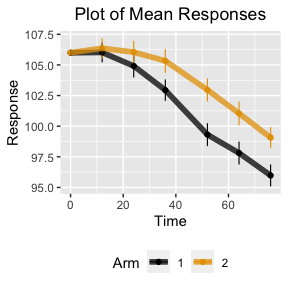### The LoE & EE tab in Alzheimer’s for the Diabetes case study (Figure S9 in publication)

plot_loe_ee (mean_list = mean_list,
ref_grp = reference_id,
stdev_vec = sigma_ar_vec,
p_loe_max = p_loe_max,
z_l_loe = z_l_loe,
z_u_loe = z_u_loe,
p_ee_max = p_ee_max,
z_l_ee = z_l_ee,
z_u_ee = z_u_ee,
up_good = up_good,
greyscale = FALSE,
static_output = TRUE)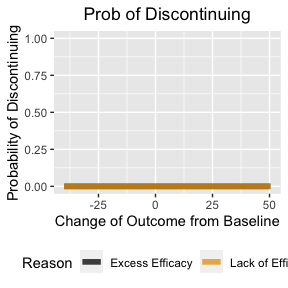### Treatment effect profiles for the three estimands in CITIES for the Alzheimer’s case study (Figure S11 in publication)

data_out = data_generator_loop(n_patient_vector,
p_loe_max,
z_l_loe,
z_u_loe,
p_ee_max,
z_l_ee,
z_u_ee,
timepoints,
pacf_list,
sigma_ar_vec,
mean_list,
beta_list,
rate_dc_ae,
prob_ae,
starting_seed_val,
reference_id,
plot_po = FALSE,
up_good,
threshold,
total_data,
covariate_df)

estimates_out = plot_estimates(data_out = data_out,
total_data = total_data,
timepoints = timepoints,
IR_display = IR_display,
reference_id = reference_id,
static_output = static_output)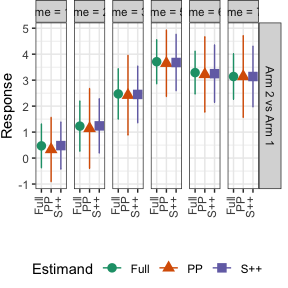### Treatment effect profiles for the three estimands in CITIES for the Alzheimer’s case study (Table 5 in publication)

display_table = estimates_out %>%
mutate(mean_se = paste0(mean, " (", round(se, 2) , ")")) %>%
dplyr::select(-se, -Arm, -mean) %>%
pivot_wider(names_from = Estimand, values_from = mean_se)
display_table[,c(1,2,5,3,4)]
## # A tibble: 6 × 5
##   name  Timepoints Full        PP          S++
##   <chr>      <dbl> <chr>       <chr>       <chr>
## 1 1             12 0.47 (0.84) 0.33 (1.23) 0.48 (0.9)
## 2 2             24 1.23 (0.96) 1.14 (1.53) 1.24 (1.04)
## 3 3             36 2.47 (0.97) 2.42 (1.53) 2.45 (1.09)
## 4 4             52 3.71 (0.85) 3.65 (1.28) 3.68 (1.08)
## 5 5             64 3.29 (0.82) 3.22 (1.45) 3.25 (1.1)
## 6 6             76 3.14 (0.88) 3.14 (1.57) 3.14 (1.16)

### Percentages of treatment discontinuations, and the corresponding ICEs in CITIES for the Alzheimer’s case study (Figure S12 in publication)

dc_out = plot_dc(data_out = data_out,
total_data = total_data,
timepoints = timepoints,
static_output = static_output)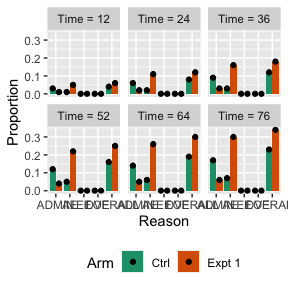### Percentages of treatment discontinuations, and the corresponding ICEs in CITIES for the Alzheimer’s case study (Table 4 in publication)

dc_out1 = dc_out %>%
ungroup() %>%
filter(Timepoints == max(timepoints),
Reason != "OVERALL") %>%
select(Arm, Reason, Value) %>%
pivot_wider(names_from = Arm,
values_from = Value) %>%
arrange(factor(Reason, levels = c("AE", "LOE", "EE", "ADMIN")))
rbind(dc_out1,c("OVERALL",colSums(dc_out1[,-1])))
## # A tibble: 5 × 3
##   Reason  Ctrl  Expt 1
##   <chr>   <chr> <chr>
## 1 AE      0.07  0.3
## 2 LOE     0     0
## 3 EE      0     0
## 5 OVERALL 0.24  0.36

### Demonstration with custom covariance and custom covariates with 3 Arms

The settings below simulates 20 datasets for a clinical trial with three arms over (0,24,48,72,96,120,144) weeks. Arm 1 has 120 subjects with a mean trajectory of (0, 0, 0, 0, 0, 0, 0, 0, 0, 0, 0, 0), while arm 2 has a mean trajectory of (0, 0.1, 0.2, 0.4, 0.6, 0.8, 1, 1.1, 1.2, 1.3, 1.4, 1.5), and finally arm 3 has a mean trajectory twice that of arm 2. The covariance structure is a randomly generated positive definite matrix, labelled Sigma. Further, a custom covariate dataframe is generated with two continuous and one binary covariates. The lack of efficacy and excess efficacy functions are shown below, alongside the behaviors of discontinuity due to adminstrative reasons and adverse events.

Note the following

• First entry for all means must be the same, i.e. the baseline mean. In this case, this is 0
• Length of all means and timepoints must be the same
• The length of each beta vector must the same as the number of covariates
• prob_ae >= rate_dc_ae per arm
• z_l_loe <= z_u_loe
• z_l_ee <= z_u_ee
• To submit a custom covariance, set sigma_ar_vec = NA and fill the pacf_list with your list of custom covariance matrices per arm.
• reference_id must be either one of the arms. For example, if you have 4 arms, then reference_id should be either 1,2,3 or 4.
p_admin_expt = 0.005

prob_ae_expt = 0.6
rate_dc_ae_expt = 0.1
prob_ae_ctrl = 0.3
rate_dc_ae_ctrl = 0.1

n_patient_expt = 120
n_patient_expt2 = 100
n_patient_ctrl = 150

mean_treatment = c(0, 0.1, 0.2, 0.4, 0.6, 0.8, 1, 1.1, 1.2, 1.3, 1.4, 1.5)
mean_treatment2 = 2*c(0, 0.1, 0.2, 0.4, 0.6, 0.8, 1,  1.1, 1.2, 1.3, 1.4, 1.5)
mean_control = rep(0, length(mean_treatment))

beta_control = c(1.25, 1.25, 1)
beta_expt = c(1.25, 1.25, 1)
beta_expt2 = c(1.25, 1.25, 1)

p_loe_max = 0.3
z_l_loe = -5
z_u_loe = -3
p_ee_max = 0.1
z_l_ee = 6
z_u_ee = 7.5
timepoints = c(0:(length(mean_treatment)-1))*24

up_good = "Up"
threshold = NA

mean_list = list(mean_control, mean_treatment, mean_treatment2)

rate_dc_ae = c(rate_dc_ae_ctrl, rate_dc_ae_expt, rate_dc_ae_expt)
prob_ae = c(prob_ae_ctrl, prob_ae_expt,prob_ae_expt)
n_patient_vector = c(n_patient_ctrl, n_patient_expt, n_patient_expt2)

sigma_ar_vec = NA

total_data = 20
reference_id = 1

starting_seed_val = 1

A = matrix(runif(length(timepoints)^2)*2-1, ncol=length(timepoints))
Sigma = t(A) %*% A
pacf_list = list(Sigma,
Sigma,
Sigma)

n_total = sum(n_patient_vector)
beta_list = list(beta_control, beta_expt, beta_expt2)

covariate_df = data.frame(continuous_1 = (rnorm(n = n_total, mean = 0, sd = 1)),
continuous_2 = (rnorm(n = n_total, mean = 0, sd = 1)),
binary_1 = rbinom(n = n_total, size = 1, prob = 0.5))

starting_seed_val = 1
static_output = TRUE
IR_display = TRUE

### The Mean Treatment Response Settings tab in CITIES for the custom covariate example

mean_out = plot_means(n_patient_vector = n_patient_vector,
timepoints = timepoints,
pacf_list = pacf_list,
sigma_ar_vec = sigma_ar_vec,
mean_list = mean_list,
beta_list = beta_list,
reference_id = reference_id,
seed_val = starting_seed_val,
total_data = total_data,
threshold = threshold,
covariate_df = covariate_df,
static_output = static_output)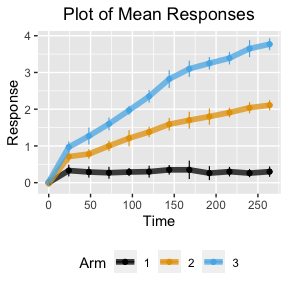### The LoE & EE tab in Alzheimer’s for the custom covariate example

plot_loe_ee (mean_list = mean_list,
ref_grp = reference_id,
stdev_vec = sigma_ar_vec,
p_loe_max = p_loe_max,
z_l_loe = z_l_loe,
z_u_loe = z_u_loe,
p_ee_max = p_ee_max,
z_l_ee = z_l_ee,
z_u_ee = z_u_ee,
up_good = up_good,
greyscale = FALSE,
static_output = TRUE)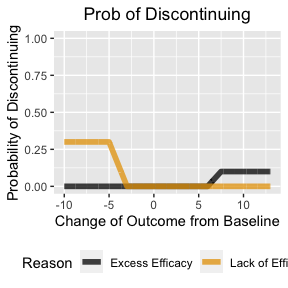### Treatment effect profiles for the four estimands in CITIES for the custom covariate example

data_out = data_generator_loop(n_patient_vector,
p_loe_max,
z_l_loe,
z_u_loe,
p_ee_max,
z_l_ee,
z_u_ee,
timepoints,
pacf_list,
sigma_ar_vec,
mean_list,
beta_list,
rate_dc_ae,
prob_ae,
starting_seed_val,
reference_id,
plot_po = FALSE,
up_good,
threshold,
total_data,
covariate_df)

estimates_out = plot_estimates(data_out = data_out,
total_data = total_data,
timepoints = timepoints,
IR_display = IR_display,
reference_id = reference_id,
static_output = static_output)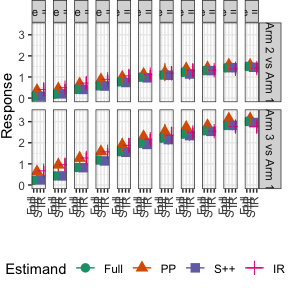estimates_out %>%
mutate(mean_se = paste0(mean, " (", round(se, 2) , ")")) %>%
dplyr::select(-se, -mean) %>%
pivot_wider(names_from = Estimand, values_from = mean_se)
## # A tibble: 22 × 7
##    Arm   name  Timepoints PP          S++       Full        IR
##    <chr> <chr>      <dbl> <chr>       <chr>       <chr>       <chr>
##  1 Arm 2 1             24 0.36 (0.28) 0.09 (0.09) 0.08 (0.1)  0.41 (0.29)
##  2 Arm 2 2             48 0.44 (0.17) 0.2 (0.13)  0.18 (0.12) 0.49 (0.18)
##  3 Arm 2 3             72 0.67 (0.26) 0.41 (0.2)  0.41 (0.15) 0.71 (0.29)
##  4 Arm 2 4             96 0.87 (0.24) 0.57 (0.14) 0.58 (0.12) 0.89 (0.21)
##  5 Arm 2 5            120 0.99 (0.28) 0.74 (0.19) 0.77 (0.15) 1.05 (0.29)
##  6 Arm 2 6            144 1.13 (0.26) 0.96 (0.18) 0.99 (0.16) 1.11 (0.21)
##  7 Arm 2 7            168 1.23 (0.41) 1.08 (0.22) 1.09 (0.16) 1.18 (0.31)
##  8 Arm 2 8            192 1.39 (0.29) 1.14 (0.26) 1.22 (0.21) 1.32 (0.29)
##  9 Arm 2 9            216 1.43 (0.32) 1.3 (0.11)  1.3 (0.1)   1.32 (0.23)
## 10 Arm 2 10           240 1.57 (0.27) 1.44 (0.21) 1.43 (0.16) 1.39 (0.27)
## # ℹ 12 more rows

### Percentages of treatment discontinuations, and the corresponding ICEs in CITIES for the custom covariate example

dc_out = plot_dc(data_out = data_out,
total_data = total_data,
timepoints = timepoints,
static_output = static_output)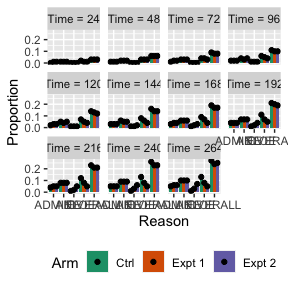dc_out1 = dc_out %>%
ungroup() %>%
filter(Timepoints == max(timepoints),
Reason != "OVERALL") %>%
select(Arm, Reason, Value) %>%
pivot_wider(names_from = Arm,
values_from = Value) %>%
arrange(factor(Reason, levels = c("AE", "LOE", "EE", "ADMIN")))
rbind(dc_out1,c("OVERALL",colSums(dc_out1[,-1])))
## # A tibble: 5 × 4
##   Reason  Ctrl  Expt 1 Expt 2
##   <chr>   <chr> <chr>    <chr>
## 1 AE      0.1   0.1      0.1
## 2 LOE     0.13  0.08     0.05
## 3 EE      0.01  0.03     0.07
## 4 ADMIN   0.05  0.06     0.06
## 5 OVERALL 0.29  0.27     0.28